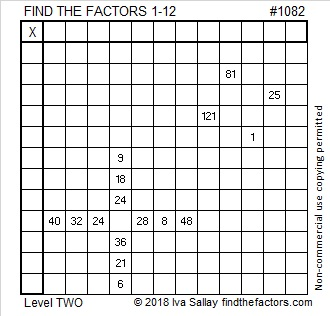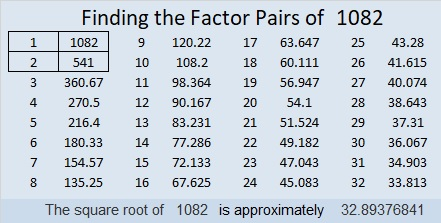# 1082 and Level 3

Write the numbers from 1 to 12 in both the first column and the top row so that this puzzle can function as a multiplication table. Do that, and you will have found all the factors 1-12 and solved the puzzle. Afterward, you or someone else can also complete the entire table, if you’d like.Print the puzzles or type the solution in this excel file: 12 factors 1080-1086

Here is a little about the number 1082:

• 1082 is a composite number.
• Prime factorization: 1082 = 2 × 541
• The exponents in the prime factorization are 1 and 1. Adding one to each and multiplying we get (1 + 1)(1 + 1) = 2 × 2 = 4. Therefore 1082 has exactly 4 factors.
• Factors of 1082: 1, 2, 541, 1082
• Factor pairs: 1082 = 1 × 1082 or 2 × 541
• 1082 has no square factors that allow its square root to be simplified. √1082 ≈ 32.8937731² + 11² = 1082

1082 is the hypotenuse of a Pythagorean triple:
682-840-1082 calculated from 2(31)(11), 31² – 11², 31² + 11²,
It is also 2 times (341-420-541)  or 2(21² – 10²), 4(21)(10), 2(21² + 10²)

1082 is palindrome 2E2 in BASE 20 (E is 14 base 10)
because 2(20²) + 14(20) + 2(1) = 1082